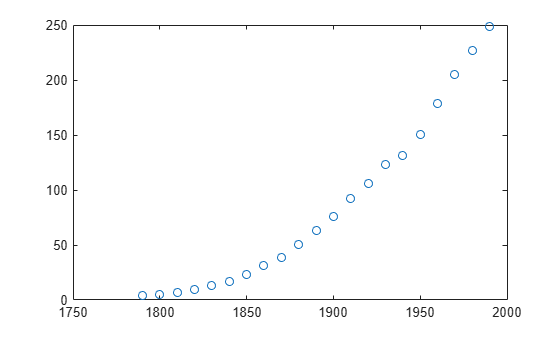# Custom Nonlinear Census Fitting

This example shows how to fit a custom equation to census data, specifying bounds, coefficients, and a problem-dependent parameter.

Load and plot the data in census.mat:

```load census plot(cdate,pop,'o') hold on```Create a fit options structure and a fittype object for the custom nonlinear model y = a(x-b)n, where a and b are coefficients and n is a problem-dependent parameter. See the fittype function page for more details on problem-dependent parameters.

```s = fitoptions('Method','NonlinearLeastSquares',... 'Lower',[0,0],... 'Upper',[Inf,max(cdate)],... 'Startpoint',[1 1]); f = fittype('a*(x-b)^n','problem','n','options',s);```

Fit the data using the fit options and a value of n = 2:

`[c2,gof2] = fit(cdate,pop,f,'problem',2)`
```c2 = General model: c2(x) = a*(x-b)^n Coefficients (with 95% confidence bounds): a = 0.006092 (0.005743, 0.006441) b = 1789 (1784, 1793) Problem parameters: n = 2 ```
```gof2 = struct with fields: sse: 246.1543 rsquare: 0.9980 dfe: 19 adjrsquare: 0.9979 rmse: 3.5994 ```

Fit the data using the fit options and a value of n = 3:

`[c3,gof3] = fit(cdate,pop,f,'problem',3)`
```c3 = General model: c3(x) = a*(x-b)^n Coefficients (with 95% confidence bounds): a = 1.359e-05 (1.245e-05, 1.474e-05) b = 1725 (1718, 1731) Problem parameters: n = 3 ```
```gof3 = struct with fields: sse: 232.0058 rsquare: 0.9981 dfe: 19 adjrsquare: 0.9980 rmse: 3.4944 ```

Plot the fit results and the data:

```plot(c2,'m') plot(c3,'c') legend( 'fit with n=2', 'fit with n=3' )```